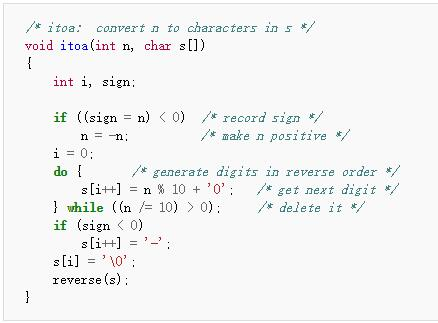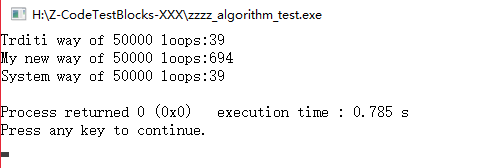# hcnak.blog

posted at 2015-12-29 13:06:53 +0000

# 几个C++数字转字符串（int转string）函数与其复杂度比较

``````error: 'itoa' was not declared in this scope
``````

``````void itoc(long n,char * num)
{
int len=0, p=n;
while(p/=10)
{
len++;
}
len++;
for(p=0;p<len;p++)
{
int x=1;
for(int t=p+1;t<len;t++)
{
x*=10;
}
num[p] = n/x + '0';
n -=( num[p] - '0' ) * x;
}
num[len] = '\0';
}
``````

## 方法二

``````inline string inttostr(int num)
{
int buf=num,x=0;
string snum="";
while(1)
{
//if(buf==1)
if(buf<10)
{
char ch=buf+'0';
snum+=ch;
break;
}
else if(buf%10)
{
buf--;
x++;
}
else if(!(buf%10) && buf !=0)
{
char ch=x+'0';
snum+=ch;
buf /= 10;
x=0;
}
}

return snum;
}
``````

## 系统的方法stdlib.h库里函数的方法用了和方法一原理上相同的方法，只不过，好吧，他这个可以判断正负。写得好简洁。`© kanch`zl AT kanchz DOT com

last updated on 2022-07-27 01:57:54 +0000